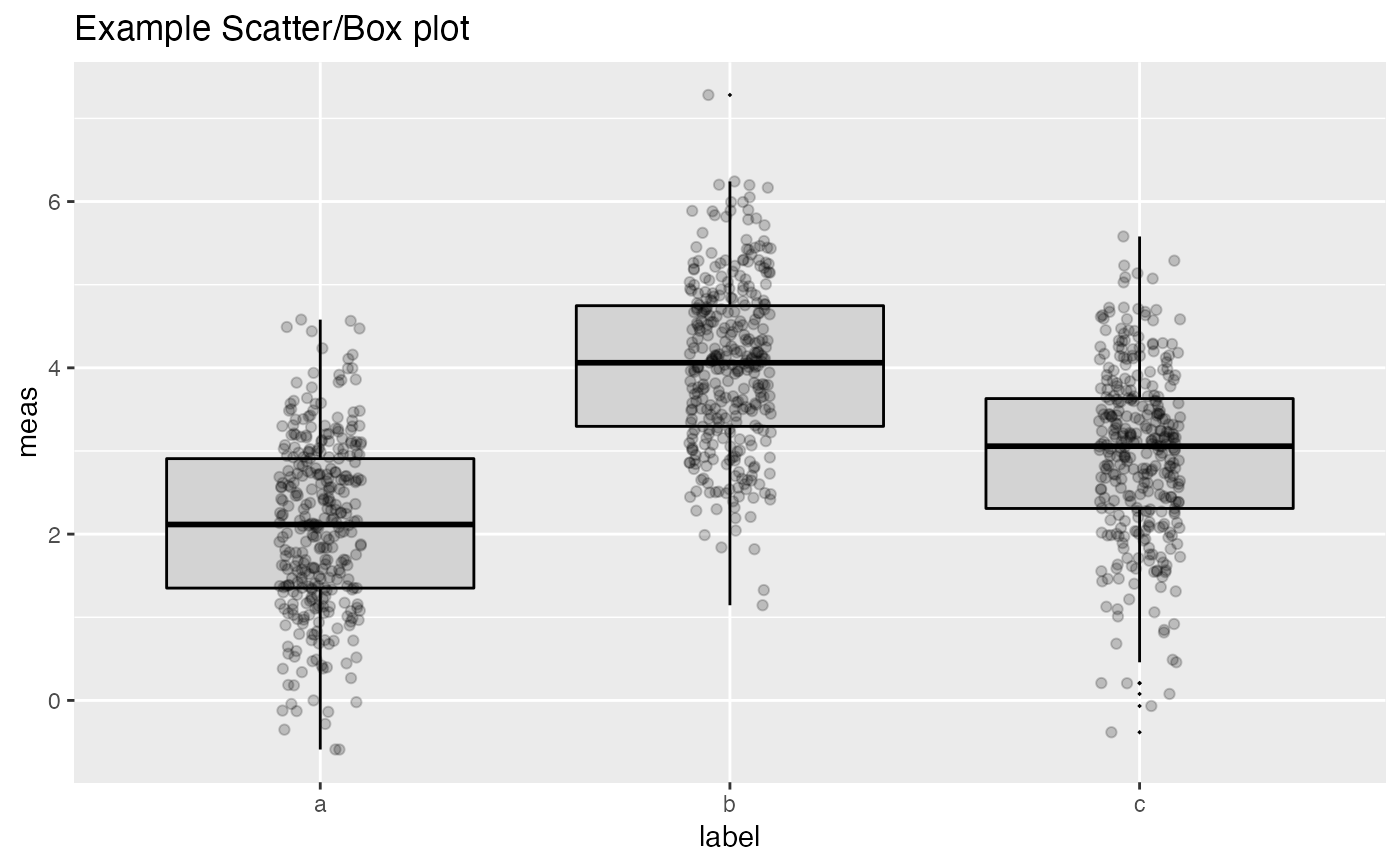Plot a boxplot with the data points superimposed.

ScatterBoxPlot(
frm,
xvar,
yvar,
title,
...,
pt_alpha = 0.3,
pt_color = "black",
box_color = "black",
box_fill = "lightgray"
)

## Arguments

frm data frame to get values from name of the independent column in frame; assumed discrete name of the continuous column in frame plot title (doesn't take additional arguments, used to force later arguments by name) transparency of points in scatter plot point color boxplot line color boxplot fill color (can be NA for no fill)

## Details

xvar is a discrete variable and yvar is a continuous variable.

ScatterBoxPlotH

## Examples


classes = c("a", "b", "c")
means = c(2, 4, 3)
names(means) = classes
label = sample(classes, size=1000, replace=TRUE)
meas = means[label] + rnorm(1000)
frm2 = data.frame(label=label,
meas = meas)
WVPlots::ScatterBoxPlot(frm2, "label", "meas", pt_alpha=0.2, title="Example Scatter/Box plot")